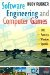# 24.1 Vectors and matrices

 The Pop Framework uses a cVector class to hold our critters' positions . Basing the cVector on real numbers helps to make our programs resolution-independent. Also the use of real numbers lets us give our simulated objects more interesting and physically realistic behaviors. We can use cVector for positions, velocities, and accelerations, and, because these vectors are based on real numbers, we can use some standard machinery from mathematics and physics. We normally define the shape of an object by a collection of vectors near the origin, and then when we want to translate or rotate the object, we imagine transforming these 'shape vectors' into some new location. Without going into much detail, let's assume that you're vaguely familiar with the notion of a matrix from mathematics “ you probably recall that a matrix is a square or rectangular array of numbers arranged as in a table, say as a four by four array. We have a certain way of 'multiplying' a matrix times a vector, and this proves useful in computer graphics for moving and rotating vectors. We will think of multiplying a chain of matrices on the left times a vector on the right. In case you're a bit foggy on matrix multiplication, you can simply think of a matrix as a transformation that can be made up of translations, rotations , scalings, and/or shears. When you see a matrix-times-vector multiplication like M*v , you can think of it as meaning something like M(v) , that is, 'the result of letting M act on v .' If View and Model are matrices, a line like Vertex = View * Model * Vertex can be read as Vertex = View(Model(Vertex)) , meaning that we are to apply first Model and then View to the Vertex . As well as using matrices to move our objects into position, we also use matrices as a way to describe the position and attitude from which the user views the simulated world. Our vector and matrix class headers and implementations live in files that are named VectorTransformation.h and VectorTransformation.cpp .Software Engineering and Computer Games
ISBN: B00406LVDU
EAN: N/A
Year: 2002
Pages: 272